# C.2 The probability density function

Bayes’ Theorem states that:(C.1)

Since we are only seeking to maximise the quantity on the left, and the denominator, termed the Bayesian evidence, is independent of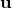, we can neglect it and replace the equality sign with a proportionality sign. Furthermore, if we assume a uniform prior, that is, we assume that we have no prior knowledge to bias us towards certain more favoured values of, thenis also a constant which can be neglected. We conclude that maximising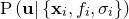is equivalent to maximising.

Since we are assuming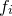to be Gaussian-distributed observations of the true function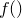, this latter probability can be written as a product of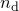Gaussian distributions: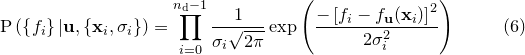(C.2)

The product in this equation can be converted into a more computationally workable sum by taking the logarithm of both sides. Since logarithms are monotonically increasing functions, maximising a probability is equivalent to maximising its logarithm. We may write the logarithm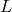ofas:(C.3)

where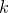is some constant which does not affect the maximisation process. It is this quantity, the familiar sum-of-square-residuals, that we numerically maximise to find our best-fitting set of parameters, which I shall refer to from here on as.